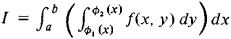# Iterated Integral

## iterated integral

[′īd·ə‚rād·əd ′int·ə·grəl]
(mathematics)
An integral over an area or volume designated to be performed by successive integrals over line segments.
McGraw-Hill Dictionary of Scientific & Technical Terms, 6E, Copyright © 2003 by The McGraw-Hill Companies, Inc.
The following article is from The Great Soviet Encyclopedia (1979). It might be outdated or ideologically biased.

## Iterated Integral

a concept of the integral calculus. Let

I = ∫∫Sf(x, y) dxdy

be the double integral of the function f(x, y) over the region S bounded by the lines x = a and x = b and the curves y = φ1(x) and y = φ2(x). If certain conditions on f(x, y), φ1(x), and φ2(x) are fulfilled, the double integral can be calculated by the formulawhere x is kept constant when the inner integral is calculated. The calculation of a double integral thus reduces to two calculations of ordinary integrals, or to the calculation of what is called an iterated integral.

Geometrically, the reduction of a double integral to an iterated integral means that the volume of a cylindroid can be calculated both by dividing it into elementary columns and by dividing it into elementary layers parallel to the yz-plane. The order of integration in the iterated integral can be changed—that is, integration may be performed first with respect to x and then with respect to y—if certain conditions are imposed on f(x, y) and S. The iterated integral is defined in a similar manner for functions of more than two variables.